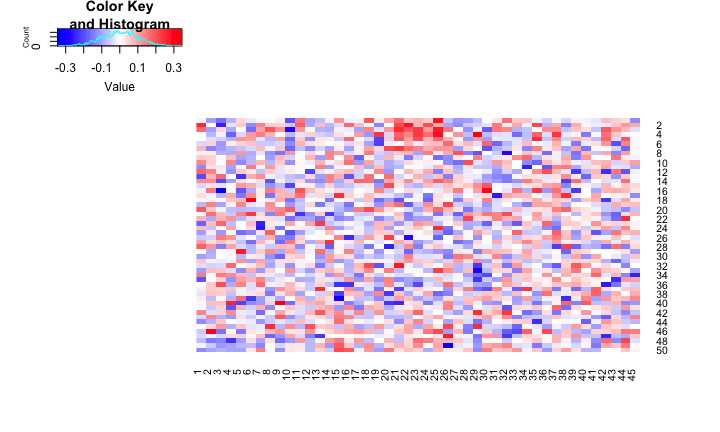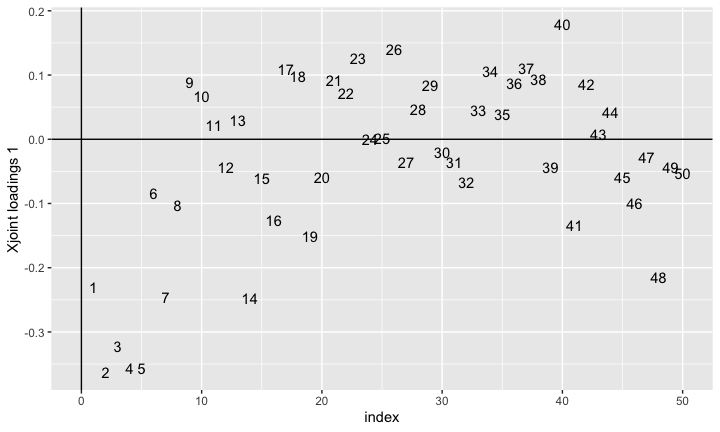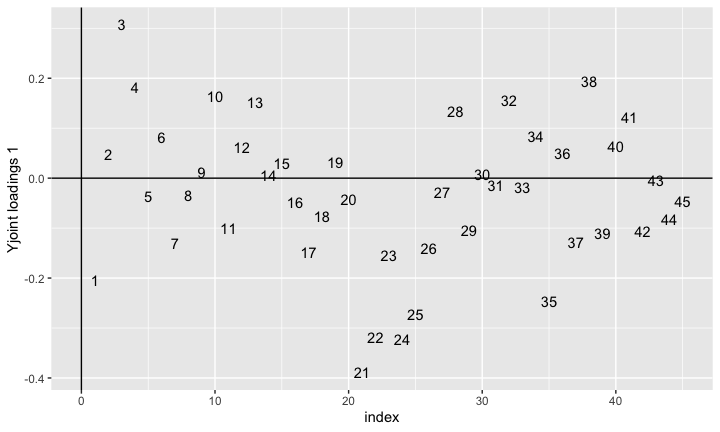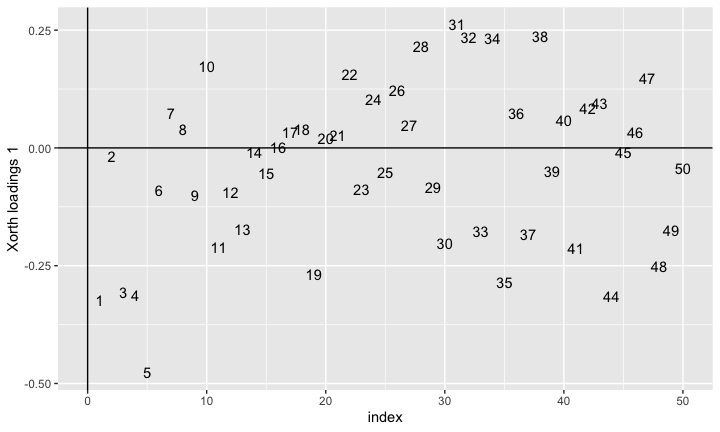# The OmicsPLS R package

Welcome to the vignette of the O2PLS package for analyzing two Omics datasets!

Here you can find examples and explanation of the input options and output objects. As always: help is always found by using the ? operator. Try to type ?OmicsPLS for an overview of the package and ?o2m for description of the main fitting function.

## Background

### The O2PLS method

The O2PLS method is proposed in (Trygg & Wold, 2003). It decomposes the variation of two datasets in three parts:

• A Joint part for $$X$$ and $$Y$$: $$TW^\top$$ and $$UC^\top$$,
• A Systematic/Specific/Orthogonal part for $$X$$ and $$Y$$: $$T_\perp W_\perp^\top$$ and $$U_\perp C_\perp^\top$$,
• A noise part for $$X$$ and $$Y$$: $$E$$ and $$F$$.

The number of columns in $$T$$, $$U$$, $$W$$ and $$C$$ are denoted by as $$n$$ and are referred to as the number of joint components. The number of columns in $$T_\perp$$ and $$W_\perp$$ are denoted by as $$n_X$$ and are referred to as the number of $$X$$-specific components. Analoguous for $$Y$$, where we use $$n_Y$$ to denote the number of $$Y$$-specific components. The relation between $$T$$ and $$U$$ makes the joint part the joint part: $$U = TB + H$$ or $$U = TB'+ H'$$. The number of components $$(n, n_X, n_Y)$$ are chosen beforehand (e.g. with Cross-Validation).

### Cross-Validation

In cross-validation (CV) one minimizes a certain measure of error over some parameters that should be determined a priori. In our case we have three parameters: $$(n, n_X, n_Y)$$. A popular measure is the prediction error $$||\hat{Y} - Y||$$, where $$\hat{Y}$$ is a prediction of $$Y$$. In our case the O2PLS method is symmetric in $$X$$ and $$Y$$, so we minimize the sum of the prediction errors: $$||\hat{X} - X||+||\hat{Y} - Y||$$. The idea is to fit O2PLS to our data $$X$$ and $$Y$$ and compute the prediction errors for a grid of values for $$n$$, $$n_X$$ and $$n_Y$$. Here $$n$$ should be a positive integer, and $$n_X$$ and $$n_Y$$ should be non-negative. The best’ integers are then the minimizers of the prediction error.

### Proposed cross-validation approach

We proposed an alternative way for choosing the number of components (el Bouhaddani, 2016). Here we construct a grid of values for $$n$$. For each $$n$$ we consider then the $$R^2$$ between $$T$$ and $$U$$ for different $$n_X$$ and $$n_Y$$. If $$T$$ and $$U$$ are contaminated with data-specific variation the $$R^2$$ will be lower. If too many specific components are removed the $$R^2$$ will again be lower. Somewhere in between is the maximum, with its maximizers $$n_X$$ and $$n_Y$$. With these two integers we now compute the prediction error for our $$n$$ that we have kept fixed. This process we repeat for each $$n$$ on the one-dimensional grid and get our maximizers. This can provide a (big) speed-up and often yields similar values for $$(n, n_X, n_Y)$$.

The easiest way is to run devtools::install_github("selbouhaddani/OmicsPLS"). If this doesn’t work, check if there is a package missing. It imports the ggplot2 and parallel package, so these should be installed first. If there still is an error, try to download the .tar or .zip (for Windows) and install offline. These two files can be found also in the selbouhaddani/ZippedPackage repository. Also feel free to send an email with the error message you are receiving.

The OmicsPLS package is loaded by running library(OmicsPLS). Maybe you get a message saying that the loadings object is masked from package::stats. This basically means that whenever you type loadings (which is generic), you’ll get the loadings.o2m variant.

## A first test case

First we generate some data

set.seed(564785412L)
X = rnorm(100) %*% t(c(rep(1,5), rep(0,45))/sqrt(5)) + # Component 1 = joint
rnorm(100) %*% t(c(rep(0,45), rep(1,5))/sqrt(5)) # Component 2 = specific
Y = X[,c(6:25, 1:5, 26:45)] # Reorder columns of X and leave out last 5
X = X + matrix(rnorm(100*50), nrow=100) # add noise
Y = Y + matrix(rnorm(100*45), nrow=100) # add noise

X = scale(X, scale=F)
Y = scale(Y, scale=F)

Now X has 100 rows and 50 columns while Y has 100 rows and 45 columns. We used two latent components in $$X$$, which are hidden in the first five and last five variables. The first five variables are also present in $$Y_{20}$$ to $$Y_{25}$$. We add noise so we do not exactly observe the latent structures.

We will use the gplots package to create heatmaps of correlations.

try(
gplots::heatmap.2(cor(X,Y), Rowv=F,Colv=F, col=gplots::bluered(100),
symm = TRUE, trace="none", dendrogram="none"),
silent = TRUE)It is difficult to see where the correlated part lies. We will try to find out with O2PLS. First we need to determine the number of components.

library(OmicsPLS)
##
## Attaching package: 'OmicsPLS'
## The following object is masked from 'package:stats':
##
##     loadings
set.seed(1221L)
crossval_o2m_adjR2(X, Y, 1:3, 0:3, 0:3, nr_folds = 2)
## Minimum is at n = 1
## Elapsed time: 0.39 sec
##        MSE n nx ny
## 1 2.036422 1  1  1
## 2 2.057468 2  3  3
## 3 2.071704 3  3  3
crossval_o2m(X, Y, 1:3, 0:3, 0:3, nr_folds = 10)
## *******************
## Elapsed time: 3.16 sec
## *******
## Minimal 10-CV error is at ax=3 ay=0 a=1
## *******
## Minimum MSE is 2.021819
## *******************

The alternative cross-validation suggests one component in all parts. The full cross-validation suggests one joint and one $$X$$-specific component. Although the full CV got it right, the alternative yielded similar answers in much less CPU time. This is partly because we use more folds, but decreasing the number of folds to two yielded unreliable results for the full CV.

We now fit the O2PLS model.

fit0 = o2m(X, Y, 1, 1, 0)
fit0
## O2PLS fit
## with 1 joint components
## and  1 orthogonal components in X
## and  0 orthogonal components in Y
## Elapsed time: 0.004 sec
summary(fit0)
##
## *** Summary of the O2PLS fit ***
##
## -  Call: o2m(X = X, Y = Y, n = 1, nx = 1, ny = 0)
##
## -  Modeled variation
## -- Total variation:
## in X: 5100.552
## in Y: 4576.141
##
## -- Joint, Orthogonal and Noise as proportions:
##
##            data X data Y
## Joint       0.040  0.054
## Orthogonal  0.042  0.000
## Noise       0.918  0.946
##
## -- Predictable variation in Y-joint part by X-joint part:
## Variation in T*B_T relative to U: 0.693
## -- Predictable variation in X-joint part by Y-joint part:
## Variation in U*B_U relative to T: 0.693
##
## -- Variances per component:
##
##          Comp 1
## X joint 206.273
## Y joint 247.776
##
##         Comp 1
## X Orth 212.371
##
##
## -  Coefficient in 'U = T B_T + H_U' model:
## -- Diagonal elements of B_T =
##  0.912

We can see that there is a lot of noise (92% and 95%), and only about 5% joint variation. However relative to this variation, 69% is predictable. To see which variables induce the joint variation, we plot the joint loadings of $$X$$ and $$Y$$.

plot(fit0)plot(fit0, "Yj")We see that more or less the first five $$X$$ variables and columns 21 to 25 of $$Y$$ have high absolute loading values.

The $$X$$-specific loadings are not recovered unfortunately, probably due to the high noise level.

plot(fit0, "Xo")`